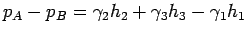# Manometer & Its Types

A Manometer by definition is a device used for measuring the pressure of a fluid by balancing it against a pre-determined column of fluid.The most common type of manometer which almost all us might have seen is sphygmomanometer. It is the device which doctors use to measure your blood pressure.

A manometer is can be used at any applicative process where the pressure of a fluid needs to be measured.

### The five basic types of manometers are:

#### 1. U-Tube Manometer:This type of manometer is U shaped, with one end open to atmosphere.It is used for measuring suction. It has the capability of measuring both positive and negative suction pressures. It utilizes the principle of specific gravities for measuring the suction pressure. This type of manometer generally has a liquid whose specific gravity is greater than that of the fluid whose suction pressure is to be measured.

Also Read: Dynamometer: Introduction, Types & Working

#### 2. Differential U-Tube Manometer:This type of manometer is slightly different from the normal U Tube manometer. Here the open end is also closed, what that means is both the ends of this type of manometer are closed.It really becomes a tool of importance where the pressure is to be measured directly.This manometer type has fluid or liquid filled at different pressures on both ends.

The equation for this is as follows: